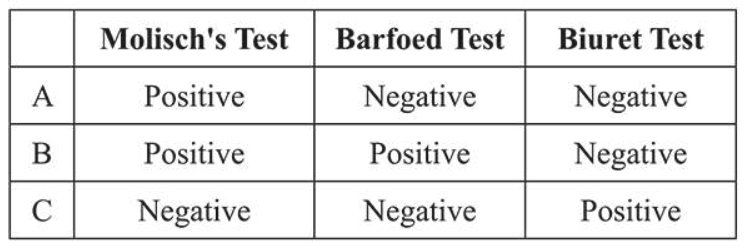# A, B and C are three biomolecules.

Question:

A, B and C are three biomolecules. The results of the tests performed on them are given below:$\mathrm{A}, \mathrm{B}$ and $\mathrm{C}$ are respectively:

1. $\mathrm{A}=$ Glucose, $\mathrm{B}=$ Fructose, $\mathrm{C}=$ Albumin

2. $\mathrm{A}=$ Lactose, $\mathrm{B}=$ Glucose, $\mathrm{C}=$ Albumin

3. $\mathrm{A}=$ Lactose, $\mathrm{B}=$ Glucose, $\mathrm{C}=$ Alanine

4. $\mathrm{A}=$ Lactose, $\mathrm{B}=$ Fructose, $\mathrm{C}=$ Alanine

Correct Option: , 2

Solution:

Molisch's test is used to check the presence of carbohydrates while Barfoed test is used for detecting the monosaccharides. Biuret test is used to detect the peptide bonds. Therefore, $\mathrm{A}$ and $\mathrm{B}$ (monosaccharide) are carbohydrates while $\mathrm{C}$ is a protein.